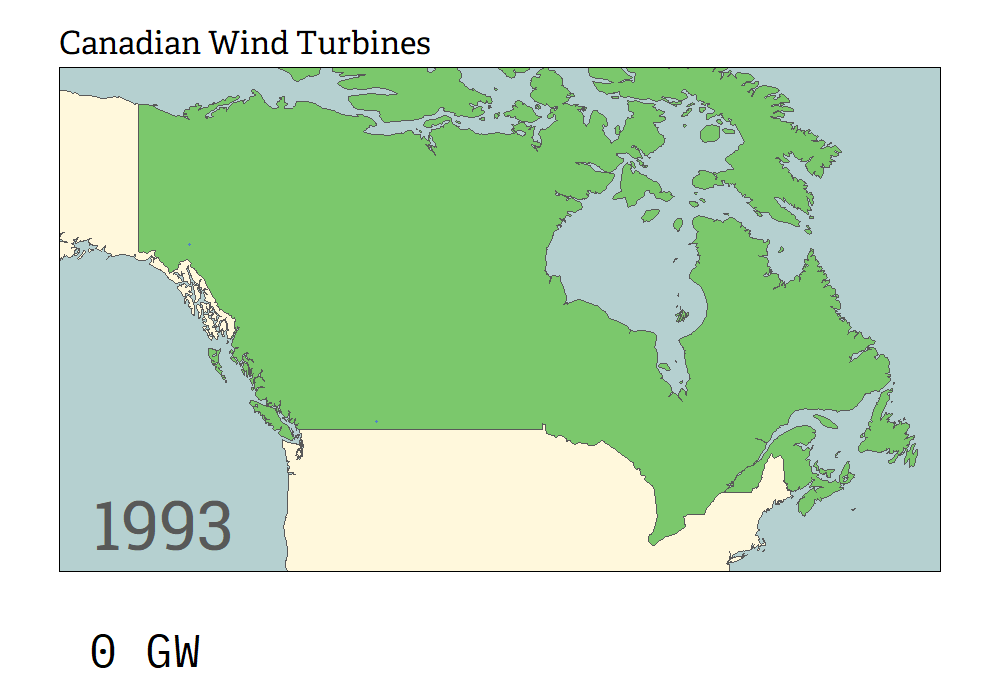# TidyTuesday 2020 week 44

Patched animation of the location and cumulative capacity of wind turbines in Canada

June Choe (University of Pennsylvania Linguistics)https://live-sas-www-ling.pantheon.sas.upenn.edu/
10-28-2020

## Visualization### Things I learned

• Using `{magick}` for animation composition, thanks to the `{gganimate}` wiki

• The very basics of working with spatial data with `{rnaturalearth}` and `{sf}`1

• A bit about color schemes for maps (I particularly love this color as a way of de-emphasizing territories in the background)

### Things to improve

• I couldn’t figure out how to add margins to the bottom, but I now realize that I could’ve just played around with `expansion()` for the y-axis of the bar animation plot.

• Image composition took a while to render, which was a bit frustrating. Need to find a way to speed that up.

## Code

Also available on github

``````library(tidyverse)
library(gganimate)
library(extrafont)

tuesdata <- tidytuesdayR::tt_load(2020, week = 44)

wind_turbine <- tuesdata\$`wind-turbine` %>%
select(
ID = objectid,
Province = province_territory,
Capacity = total_project_capacity_mw,
Diameter = rotor_diameter_m,
Height = hub_height_m,
Year = commissioning_date,
Lat = latitude,
Lon = longitude
) %>%
arrange(Year, -Diameter) %>%
mutate(
Year = as.integer(str_match(Year, "^\\d{4}")[,1])
)

ne_map <- rnaturalearth::ne_countries(scale='medium', returnclass = 'sf')

turbine_anim <- wind_turbine %>%
ggplot() +
geom_rect(
aes(xmin = -150, xmax = -50, ymin = 40, ymax = 72),
fill = "#B6D0D1"
) +
geom_sf(
show.legend = FALSE,
) +
scale_fill_identity() +
geom_point(
aes(Lon, Lat, group = ID, size = Capacity),
show.legend = FALSE, alpha = 0.5, color = "#3C59FF"
) +
geom_text(
aes(x = -138, y = 43, label = as.character(Year)),
size = 24, color = "grey35", family = "Roboto Slab"
) +
geom_rect(
aes(xmin = -150, xmax = -50, ymin = 40, ymax = 72),
fill = "transparent", color = "black"
) +
coord_sf(
xlim = c(-150, -50),
ylim = c(40, 72),
expand = FALSE,
clip = "on"
) +
theme_void() +
theme(
plot.title = element_text(family = "Adelle", s),
plot.margin = margin(1, 1, 1, 1, "cm")
) +
transition_reveal(Year)

animate(turbine_anim, width = 1000, height = 600, nframes = 100)

capacity_data <- wind_turbine %>%
group_by(Year) %>%
summarize(
Capacity = sum(Capacity),
.groups = 'drop'
) %>%
mutate(
Capacity = accumulate(Capacity, sum),
width = (Capacity/max(Capacity)) * 70
)

capacity_anim <- capacity_data %>%
ggplot(aes(x = 1, y = Capacity)) +
geom_col(
fill = "#3C59FF",
) +
geom_text(
aes(label = paste(as.character(round(Capacity * 0.001)), "GW")),
hjust = -.2,
family = "IBM Plex Mono"
) +
scale_y_continuous(expand = expansion(c(.1, .4))) +
coord_flip() +
theme_void() +
transition_states(Year)

animate(capacity_anim, res = 300, width = 1000, height = 100, nframes = 100)

library(magick)